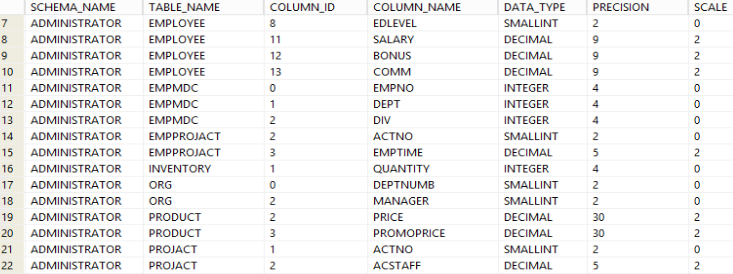## Find all numeric columns in Db2 database

Article for:

Numeric in Db2 database are columns with the following data types: smallint, integer/int, bigint, decimal/numeric, real, double, decfloat.

The query below lists all columns with numeric data types.

## Query

``````select c.tabschema as schema_name,
c.tabname as table_name,
c.colno as column_id,
c.colname as column_name,
c.typename as data_type,
c.length as precision,
c.scale
from syscat.columns c
join syscat.tables t on c.tabschema = t.tabschema
and c.tabname = t.tabname
and t.type = 'T'
where c.typename in ('SMALLINT', 'INTEGER', 'BIGINT',
'DOUBLE', 'REAL', 'DECIMAL', 'DECFLOAT')
and c.tabschema not like 'SYS%'
order by schema_name,
table_name,
column_id;
``````

## Columns

• schema_name - name of the schema
• table_name - name of the table
• column_id - column position in table
• column_name - name of the column
• data_type - type of data
• precision - precision of the numeric type
• scale - scale of numeric column

## Rows

• One row represents one column with a numeric data type
• Scope of rows: all columns containing numeric data types in the database
• Ordered by database name and table name

## Sample results